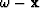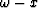# Numerical analysis ofmigration

Lewei Mo

Author has no known email address

# ABSTRACT

For the constant velocity 15-degree space-frequency () time migration algorithm, the parabolic approximation of the exact one-way wave equation causes undermigration, and the Crank-Nicolson numerical method that is used to solve the diffraction equation causes overmigration. However, the modified wave equation that the numerical solution actually satisfies for the diffraction equation shows that the trace spacing interval plays a larger role than the depth step size of downward continuation in determining the accuracy of the numerical solution.